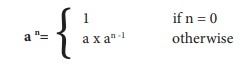Home | | Computer Science 11th std | Choose the correct answer

## Chapter: 11th Computer Science : Chapter 8 : Iteration and recursion

Multiple choice questions with answers / choose the correct answer with answers - Computer Science Book back 1 mark questions and answers - Computer Science: Algorithmic Problem Solving : Iteration and recursion

Computer Science: Algorithmic Problem Solving

Iteration and recursion

Evaluation

Part I

1. A loop invariant need not be true

(a) at the start of the loop.

(b) at the start of each iteration

(c) at the end of each iteration

(d) at the start of the algorithm

2. We wish to cover a chessboard with dominoes, the number of black squares and the number of white squares covered by dominoes, respectively, placing a domino can bemodeled by

(a) b := b + 2

(b) w := w + 2

(c) b, w := b+1, w+1

(d) b := w

3. If m x a + n x b is an invariant for the assignment a, b : = a + 8, b + 7, the values of m and n are

(a) m = 8, n = 7

(b) m = 7, n = -8

(c) m = 7, n = 8

(d) m = 8, n = -7

Solution :

7a-8b

= 7(a+8) - 8 (b+7)

= 7a + 56 - 8b -56 = 7a - 8b.

4. Which of the following is not an invariant of the assignment? m, n := m+2, n+3

(a) m mod 2

(b) n mod 3

(c) 3 X m - 2 X n

(d) 2 X m - 3 X n

5. If Fibonacci number is defined recursively asto evaluate F(4), how many times F() is applied?

(a) 3

(b) 4

(c) 8

(d) 9

6. Using this recursive definitionhow many multiplications are needed to calculate a10?

(a) 11

(b) 10

(c) 9

(d) 8

Solution :

an = a × an -1

a10 = a × a9      (1)

=a × a × a8         (2)

= a × a × a × a7         (3)

= a × a × a × a × a6         (4)

= a × a × a × a × a × a5         (5)

= a × a × a × a × a × a × a4         (6)

= a × a × a × a × a × a × a × a3         (7)

= a × a × a × a × a × a × a × a × a2         (8)

= a × a × a × a × a × a × a × a × a × a         (9)

Tags : Iteration and recursion | Computer Science , 11th Computer Science : Chapter 8 : Iteration and recursion
Study Material, Lecturing Notes, Assignment, Reference, Wiki description explanation, brief detail
11th Computer Science : Chapter 8 : Iteration and recursion : Choose the correct answer | Iteration and recursion | Computer Science